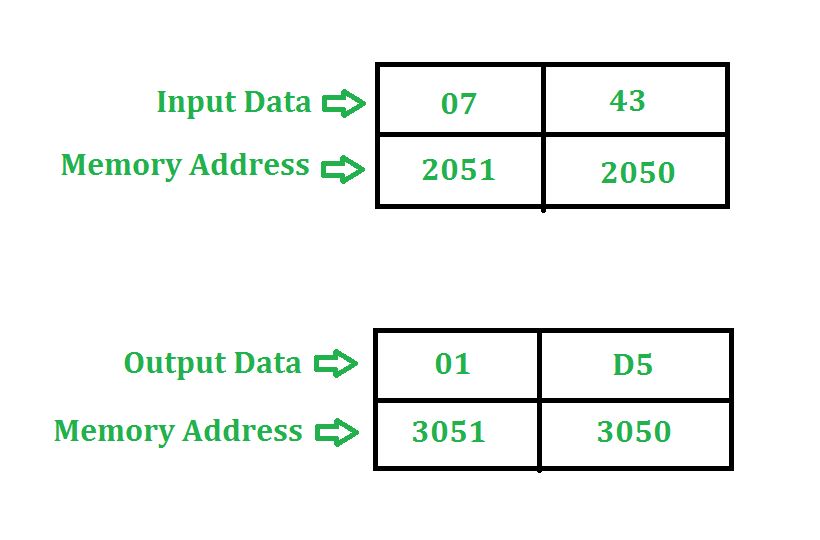Open in App
Not now

# 8085 program to multiply two 8 bit numbers

• Difficulty Level : Medium
• Last Updated : 14 Jun, 2021

Problem – Multiply two 8 bit numbers stored at address 2050 and 2051. Result is stored at address 3050 and 3051. Starting address of program is taken as 2000.

Example –Algorithm –

1. We are taking adding the number 43 seven(7) times in this example.
2. As the multiplication of two 8 bit numbers can be maximum of 16 bits so we need register pair to store the result.

Program –

Explanation – Registers used: A, H, L, C, D, E

1. LHLD 2050 loads content of 2051 in H and content of 2050 in L
2. XCHG exchanges contents of H with D and contents of L with E
3. MOV C, D copies content of D in C
4. MVI D 00 assigns 00 to D
5. LXI H 0000 assigns 00 to H and 00 to L
6. DAD D adds HL and DE and assigns the result to HL
7. DCR C decrements C by 1
8. JNZ 200A jumps program counter to 200A if zero flag = 0
9. SHLD stores value of H at memory location 3051 and L at 3050
10. HLT stops executing the program and halts any further execution

Another approach:

We can do multiplication of two 8-bit numbers without using DAD and XCHG command.

Program-

Explanation –Registers A, H, L, C, B are used for general purpose.

1. LXI H, 2050 will load the HL pair register with the address 2050 of memory location.

2. MOV B, M  copies the content of memory into register B.

3. INX H will increment the address of HL pair by one and make it 2051H.

4. MOV C,M copies the content of memory into register C.

5. MVI A,00H assign 00 to A.

6. top: ADD B add the content of accumulator with register B and store the result in accumulator.

7. DCR C decrement the register C.

8. JNZ TOP jumps on top till C doesn’t becomes 0.

9. INX H  will increment the address of HL pair by one and make it 2052H.

10. MOV M,A copies the content of  A which is our answer to register M.

11. HLT stops executing the program and halts any further execution.

My Personal Notes arrow_drop_up
Related Articles